## Who is Julia, what is she?

• Started at MIT 2010, mainly seen then as scientific language
• Open-source in 2012 where it's flexibility lead to application in many other fields
• Consists of a Base which is written in Julia
• Base contains all of "numpy", part of "scipy"
• Much of rest is implemented in packages:
• StatsBase
• MultivariateStats
• KernelDensity / KernelEstimator
• Dataframes
• RDatasets
• GLM
• Lora / MCMC
• Clustering

## Groups:

### JuliaQuant group includes packages such as :

• TimeSeries
• MarketData
• MarketTechnicals
• Quandl
• Ito
• GARCH

## Why is Julia special?

• Julia is written in Julia, down to it's cotton socks
• Uses LLVM / JIT compilation
• Homoiconic design: runtime macros
• Code is uncluttered
• Analysts => developers while remaining within Julia
• Written with parallelism in mind
• Can connect with modules / libraries in other programming languages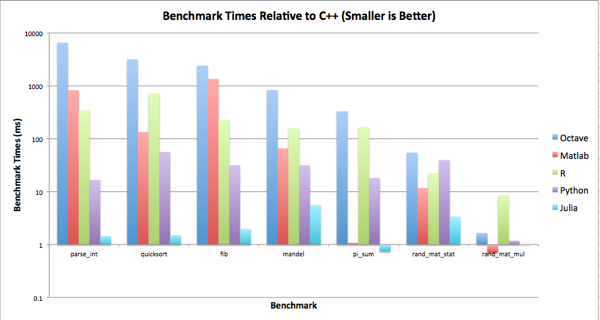## Interoperability with other languages

• C / Fortran : Built-in to BASE with NO overhead
• Java : JavaCall.jl
• C++ : Cpp.jl, Cxx.jl
• R : RIF.jl, RCall.jl
• Python: PyCall.jl, PyPlot.jl, IJulia.jl, PyJulia.jl
• MATLAB: Matlab.jl, MATLABCluster.jl

In :
using PyCall, PyPlot

In :
using PyCall

@pyimport numpy as np
@pyimport numpy.random as nr

S0 = 100.0;
K = 105.0;
T = 1.0;
r = 0.05;
σ = 0.2;

In :
N = 100000;
w = nr.standard_normal(N);

ST = S0*np.exp((r - 0.5*σ*σ)*T + σ*np.sqrt(T)*w);
hT = np.maximum(ST - K, 0);

C0 = np.exp(-r*T) * np.sum(hT)/N;
@printf "Price of %s option is %6.3f\n" "Call" C0

Price of Call option is  7.987


In :
macroexpand(:(@printf "Price of %s option is %6.3f\n" "Call" C0))

Out:
quote
#1176#out = Base.Printf.STDOUT
#1177###x#8528 = "Call"
#1178###x#8529 = C0
local #1183#neg, #1182#pt, #1181#len, #1175#exp, #1179#do_out, #1180#args
Base.Printf.write(#1176#out,"Price of ")
begin
Base.Printf.print(#1176#out,#1177###x#8528)
end
Base.Printf.write(#1176#out," option is ")
if Base.Printf.isfinite(#1178###x#8529)
(#1179#do_out,#1180#args) = Base.Printf.fix_dec(#1176#out,#1178###x#8529,"",6,3,'f')
if #1179#do_out
(#1181#len,#1182#pt,#1183#neg) = #1180#args
(Base.Printf.-(Base.Printf.-(2,if (#1182#pt Base.Printf.> 0)
#1182#pt
else
1
end),#1183#neg) Base.Printf.> 0) && Base.Printf.write(#1176#out,' ')
#1183#neg && Base.Printf.write(#1176#out,'-')
Base.Printf.print_fixed(#1176#out,3,#1182#pt,#1181#len)
end
else
Base.Printf.write(#1176#out,begin  # printf.jl, line 143:
if Base.Printf.isnan(#1178###x#8529)
"   NaN"
else
if (#1178###x#8529 Base.Printf.< 0)
"  -Inf"
else
"   Inf"
end
end
end)
end
Base.Printf.write(#1176#out,'\n')
Base.Printf.nothing
end

In :
using PyPlot

@pyimport scipy.interpolate as spi
x = linspace(0,10,10);
y = x .* exp(-0.5x) .* sin(x);
f1 = spi.interp1d(x, y);
f2 = spi.interp1d(x, y, kind="cubic");

In :
xx = linspace(0,10,40);
y1 = pycall(f1,PyAny,xx);
y2 = pycall(f2,PyAny,xx);

plot(x,y,"o",xx,y1,"-", xx, y2,"--");
legend(["data", "linear", "cubic"], loc="best");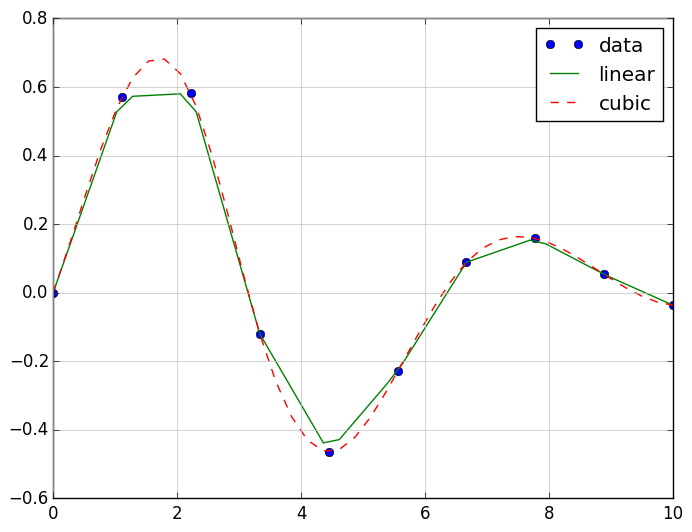In []:
In :
using PyPlot

T = 100;
S0 = 100;
dt = 0.01;
v = 0.2;
r = 0.05;
q = 0.0;

x = linspace(1,T);
S = zeros(Float64,T)
S = S0;

iseed = ccall( (:clock, "libc"), Int32, ());
srand(iseed);

dW = randn(T)*sqrt(dt);
[ S[t] = S[t-1] * (1 + (r - q - 0.5*v*v)*dt + v*dW[t] + 0.5*v*v*dW[t]*dW[t]) for t=2:T ]

plot(x,S)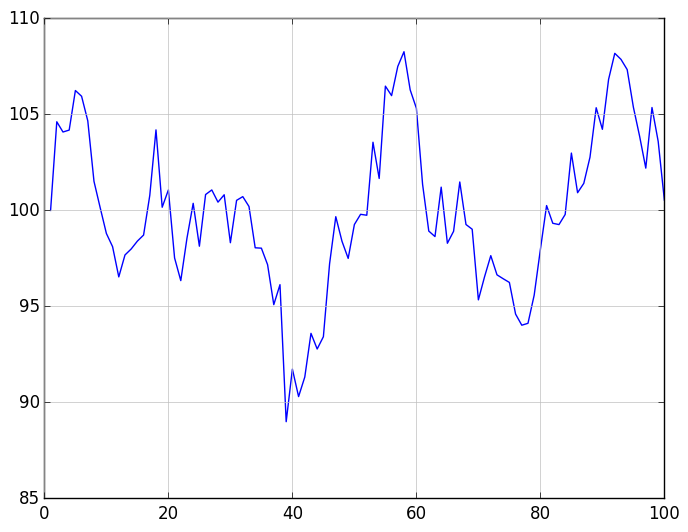Out:
1-element Array{Any,1}:
PyObject <matplotlib.lines.Line2D object at 0x1208ab910>

In :
function asianOpt(N = 10000; S0=100.0, K=100.0)

# European Asian option.
# Euler and Milstein discretization for Black-Scholes.

r   = 0.05;     # Risk free rate
q   = 0.0;      # Dividend yield
v   = 0.2;      # Volatility
tma = 0.25;     # Time to maturity

T = 100;         # Number of time steps
dt = tma/T;      # Time increment

S = zeros(Float64,T);
A = zeros(Float64,N);

for n = 1:N
S = S0
dW = randn(T)*sqrt(dt);
for t = 2:T
z0 = (r - q - 0.5*v*v)*S[t-1]*dt;
z1 = v*S[t-1]*dW[t];
z2 = 0.5*v*v*S[t-1]*dW[t]*dW[t];
S[t] = S[t-1] + z0 + z1 + z2;
end
A[n] = mean(S);
end

# Define the payoff and calculate price

P = zeros(Float64,N);
[ P[n] = max(A[n] - K, 0) for n = 1:N ];
price = exp(-r*tma)*mean(P);

end

Out:
asianOpt (generic function with 2 methods)

In :
@printf "Option Price: %10.4f\n\n" asianOpt(K = 102.0);

Option Price:     1.6600


In :
runs = 1000000
tm = @elapsed asianOpt(runs, K=102.0)
@printf "Elapsed time for %d runs is %7.4f sec.\n\n" runs tm

Elapsed time for 1000000 runs is  3.5874 sec.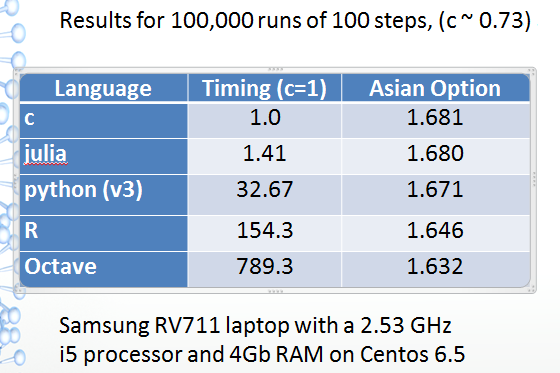In :
macroexpand(:(@elapsed asianOpt(runs, K=102.0)))

Out:
quote  # util.jl, line 68:
local #1206#t0 = Base.time_ns() # line 69:
local #1207#val = asianOpt(runs,K=102.0) # line 70:
Base./(Base.-(Base.time_ns(),#1206#t0),1.0e9)
end

In []:
macroexpand(:(@printf "Elapsed time for %d simulations is %7.3f sec.\n\n" runs tm ))

In []:### PyJulia adds a Python 'julia' module

Get source from github and use setup.py

Pkg.add("pyjulia")

cd ~/.julia/v0.3/pyjulia
python setup.py install


### Speeding up Python execution times

$python Python 2.7.9 |Anaconda 2.1.0 (x86_64) [GCC 4.2.1 (Apple Inc. build 5577)] on darwin >>> import time as tm >>> start = tm.time() >>> asianOpt(1000000,K=102.0) 1.6409 >>> print 'It took', tm.time()-start, 'seconds.' It took 77.332 seconds. >>> import julia >>> jl = julia.Julia() >>> print jl.bessely0(1.5) 0.3824489237977 >>> import numpy as np >>> print np.sin(0.5) * jl.bessely0(1.5) 0.1833557812803 >>> jl.require("asian-opt") >>> jl.asianOpt() AttributeError: 'Julia' object has no attribute 'asianOpt' >>> jl.eval("asianOpt(1000000,K=102.0)") 1.6775348594420794 >>> jl.eval("@elapsed asianOpt(1000000,K=102.0)") 3.146388532  In []:$ more myfinx.py

import datetime
import numpy as np
import matplotlib.colors as colors
import matplotlib.finance as finance
import matplotlib.dates as mdates
import matplotlib.ticker as mticker
import matplotlib.mlab as mlab
import matplotlib.pyplot as plt
import matplotlib.font_manager as font_manager

def moving_average(x, n, type='simple'):
"""
compute an n period moving average.
type is 'simple' | 'exponential'
"""

def relative_strength(prices, n=14):
"""
compute the n period relative strength indicator
http://stockcharts.com/school/doku.php?id=chart_school:glossary_r#relativestrengthindex
http://www.investopedia.com/terms/r/rsi.asp
"""

def moving_average_convergence(x, nslow=26, nfast=12):
"""
compute the MACD (Moving Average Convergence/Divergence) using a fast and slow exponential moving avg'
return value is emaslow, emafast, macd which are len(x) arrays
"""

def run(ticker):
startdate = datetime.date(2000,1,1)
today = enddate = datetime.date.today()
............
............
............
plt.show()

In :
using PyCall

@pyimport myfinx as fx
fx.run("MSFT")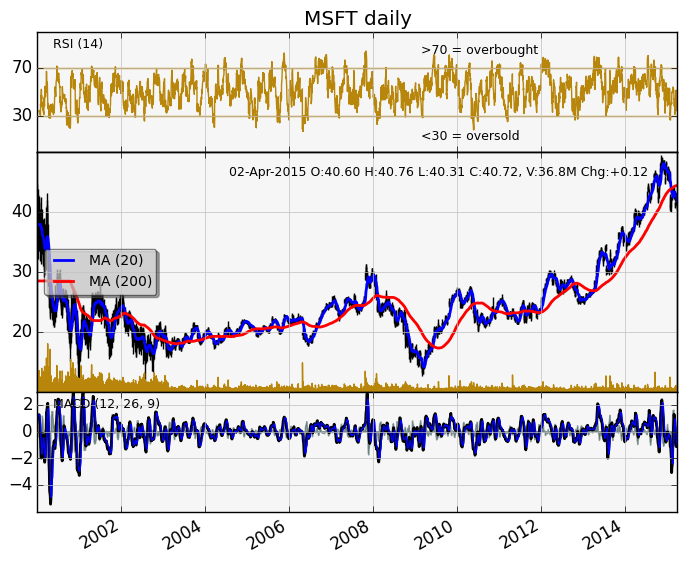In []: